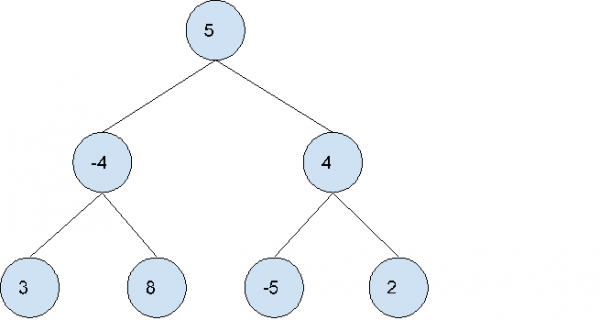# Find largest subtree sum in a tree in C++

In this problem, we are given a binary tree. Our task is to find the largest subtree sum in a tree.

Problem Description: The  binary tree consists of positive as well as negative values. And we need to find the subtree that has the maximum sum of nodes.

Let’s take an example to understand the problem,Output: 13

Explanation:

The sum of left-subtree is 7
The sum of right-subtree is 1
The sum of tree is 13

## Solution Approach

To solve the problem, we will do the post-order traversal. Calculate sum of nodes left subtree and right subtree. For current node, check if the sum of nodes of current node is greater than sum of left or right subtree. For each node from leaf to root find the maximum sum.

## Example

Live Demo

#include <iostream>
using namespace std;

struct Node {
int key;
Node *left, *right;
};

Node* newNode(int key) {

Node* temp = new Node;
temp->key = key;
temp->left = temp->right = NULL;
return temp;
}

int calcSumTreeSumRec(Node* root, int&amp; ans) {

if (root == NULL)
return 0;
int currSum = root->key + calcSumTreeSumRec(root->left, ans)+ calcSumTreeSumRec(root->right, ans);
ans = max(ans, currSum);

return currSum;
}

int calcMaxSubTreeSum(Node* root)
{
if (root == NULL)
return 0;
int ans = -100;
calcSumTreeSumRec(root, ans);
return ans;
}

int main() {

Node* root = newNode(5);
root->left = newNode(-4);
root->right = newNode(4);
root->left->left = newNode(3);
root->left->right = newNode(8);
root->right->left = newNode(-5);
root->right->right = newNode(2);

cout<<"The largest subtree sum is "<<calcMaxSubTreeSum(root);
return 0;
}

## Output

The largest subtree sum is 13